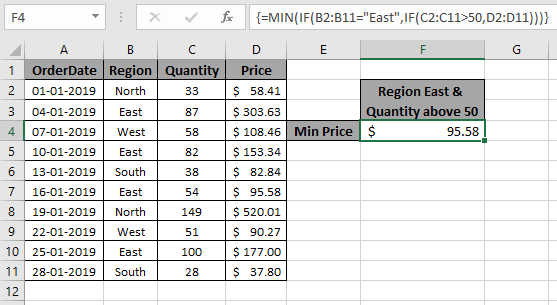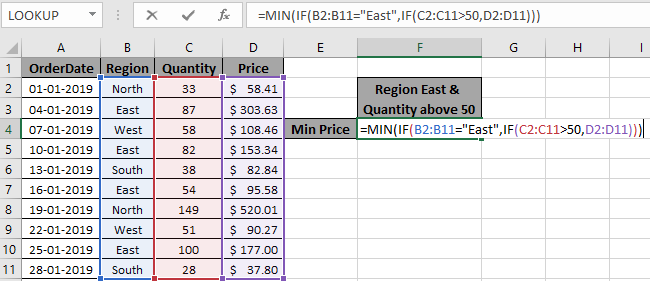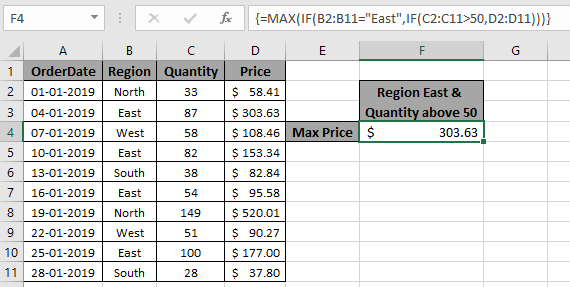# How to find minimum value with multiple criteria in ExcelIn this article, we will learn about how to find the minimum value if it matches multiple conditions in Excel.

Scenario:

When working with long ranges of data, we need to find the minimum value among the range where more than one condition is matching. In simple words finding out the minimum value using Excel IF function. IF function returns True or False and MIN function looks for the minimum value from the corresponding array.

Syntax to find min with multiple criteria

{=MIN (IF (Criteria1=match1),IF(Criteria2=match2, range_min))}

Note: Use Ctrl + Shift + Enter when working with arrays or ranges in Excel. This will generate Curly Braces on the formula by default. DO NOT try to hard code curly braces characters.

Example:

All of these might be confusing to understand. So, let's test this formula via running it on the example shown below. Here we will perform the formula over values with given criteria.

Use the formula:

{ =MIN( IF( B2:B11="East", IF( C2:C11 > 50 , D2:D11 )))}

criteria 1 is price must be from the region "East"

criteria 2 is price where quantity is greater than 50.

Explanation:

1. IF( C2:C11 > 50 , D2:D11 ) returns an array of FALSE values and price values where quantity is greater than 50.

{ FALSE ; 303.63 ; 108.46 ; 153.34 ; FALSE ; 95.58 ; 520.01 ; 90.27 ; 177 ; FALSE }

1. IF( B2:B11="East", IF( C2:C11 > 50 , D2:D11 )) returns an array of remaining price values where region is East.
2. MIN function finds the minimum value from the returned array and that would be the required PRICE value.Here we matched the range (B2:B11) with value "East" and quantity (C2:C11) greater than 50, which returns the minimum from the price range (D2:D11). Press Enter to get the minimum of the rangeAs you can see we have the MIN value, if criteria match from the range of values.

Here are some observational notes using the above formula.

Notes:

1. The formula returns the min value from the range.
2. Use MINIFS function in MS Excel 365 version to get the minimum value from data having multiple criteria. Learn more about MINIFS function here.
3. Named range in the formula be used with correct keywords.

Hope this article about How to Find the Minimum with multiple criteria in Excel is explanatory. Find more articles on reference formulas here. If you liked our blogs, share it with your fristarts on Facebook. And also you can follow us on Twitter and Facebook. We would love to hear from you, do let us know how we can improve, complement or innovate our work and make it better for you. Write to us at info@exceltip.com.

Related Articles

IF with AND function : Implementation of logic IF function with AND function to extract results having criteria in Excel.

IF with OR function : Implementation of logic IF function with OR function to extract results having criteria in excel data.

How to use nested IF function : nested IF function operates on data having multiple criteria. The use of repeated IF function is nested IF excel formula.

SUMIFS using AND-OR logic : Get the sum of numbers having multiple criteria applied using logic AND-OR excel function.

Minimum value using IF function : Get the minimum value using the excel IF function and MIN function on array data.

How to use wildcards in excel : Count cells matching phrases using the wildcards in excel

Popular Articles

50 Excel Shortcut to Increase Your Productivity : Get faster at your task. These 50 shortcuts will make you work even faster on Excel.

The VLOOKUP Function in Excel : This is one of the most used and popular functions of excel that is used to lookup value from different ranges and sheets.

COUNTIF in Excel 2016 : Count values with conditions using this amazing function. You don't need to filter your data to count specific values. Countif function is essential to prepare your dashboard.

How to Use SUMIF Function in Excel : This is another dashboard essential function. This helps you sum up values on specific conditions.

Terms and Conditions of use

The applications/code on this site are distributed as is and without warranties or liability. In no event shall the owner of the copyrights, or the authors of the applications/code be liable for any loss of profit, any problems or any damage resulting from the use or evaluation of the applications/code.